Previous: List of symbols Up: List of symbols Next: Greek symbols
This is an automatically generated documentation by LaTeX2HTML utility. In case of any issue, please, contact us at info@cfdsupport.com.

## Latin symbols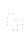skin friction coefficient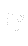specific heat capacity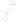diffusion coefficient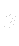gravity force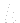internal energy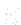surface normal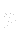pressure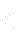heat flux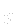source term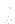time in general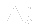time time step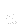velocity components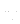velocity vector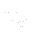friction velocity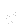velocity components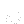velocity components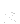space coordinates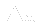space step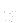vector of space coordinates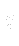space coordinates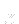space coordinates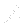general variable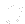general constant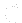concentration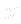test case number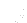diameter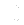cell volume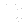rate-of-deformation tensor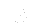Deborah number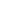vector of viscous fluxes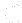vector of viscous fluxes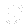spring constant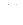vector of viscous fluxes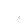characteristic length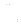velocity gradient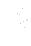Péclet number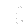characteristic radius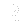vector of viscous fluxes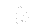Reynolds number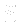vector of viscous fluxes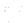physical time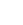vector of viscous fluxes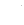stress tensor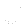mean velocity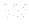vector of unknowns anti-symmetrical part of velocity gradient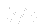Weissenberg number